# Subordination principle

One of the forms of the Lindelöf principle, which employs the concept of subordination of functions. Letbe any function regular in the disc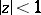and satisfying the conditions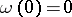,in; let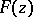be a meromorphic function in. If the functionhas the form, thenis called subordinate to the functionin the disc, whileis called the subordinating function. This subordination relation is denoted by. In the simplest case whereis a univalent function in, this relation simply means that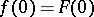and thatdoes not take any values in the discthat are not taken there by. The following basic theorems apply.

## Contents

### Theorem 1.

Let the functionbe meromorphic in the discand map it on the Riemann surface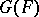. Let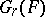be the part ofcorresponding to,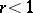. If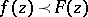, then the values ofin(under analytic continuation from) lie in, and the boundary points inare obtained only for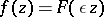,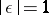.

### Theorem 2.

Ifand ifis regular in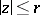,, then setting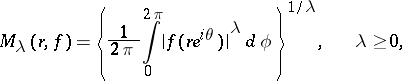one has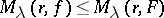,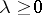,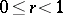, .

### Theorem 3.

Ifandis regular at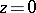, then for the coefficients of the expansions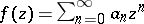,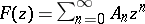one has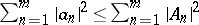,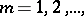.

The general theory of subordination is useful in considering the set of values taken or produced by an analytic function. The subordination relation can be used in two different ways. First, one can start from a given functionand examine the behaviour of allsubordinate to. Ifis completely known, then the regionin which the values oflie is also known (Theorem 1) as well as an upper bound on the integral means(Theorem 2). If alsois regular at, there are upper bounds for the coefficients of(Theorem 3). Secondly, one can consider a functionthat is meromorphic in the discand whose properties imply that it cannot be subordinate to a given functionin. If here, for example, is univalent, thennecessarily takes values outsidein. These ideas of using the subordination relation illustrate the subordination principle and can be extended to multiply-connected domains .